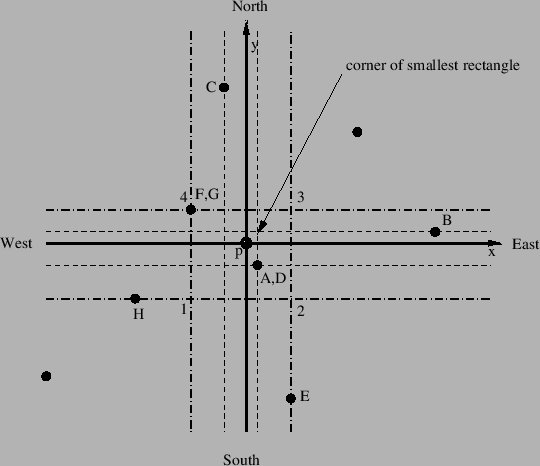### Nearest point algorithm

The centers of gravity of the linear tetrahedra form a cloud of points. One of the key parts of the algorithm is the fast selection of the n closest points to a given new location in which interpolation is requested. The procedure is illustrated in two dimensions in Figure 196.The point on the cross section of the two global axes p is the point in which the interpolation is to be performed. The black circles symbolize the point cloud. Assume we would like to know the closest 3 points. The points in the cloud are available in two sorted arrays, one sorted in the global x-direction and one in the global y-direction. First, the location of the point p in these two arrays is determined using the routine ident.f. Then, a rectangle is expanded about p reaching in each direction east, north, west and south until the next point in the cloud is attained. This is symbolized with the simple dashed lines and the points are denoted A, B, C and D (A and D coincide). Then, the distance from p to these points is calculated, sorted, and stored in an array. The size of this array is the number of closest neighbors requested, so in our case only the smallest three distances are kept.

Next, the rectangle is expanded in each direction until the next cloud point is reached, denoded by E, F, G and H. Again, the distance from p to these points is calculated, stored in the distance array, sorted and the lowest three values are kept (only the three closest points are needed). Now, this procedure is repeated until the smallest distance from p to one of the corner points of the rectangle (symbolized by 1, 2, 3 and 4 for the largest rectangle shown in the figure) exceeds the largest value in the distance array. Then we know that we have found the closest three neighboring points.

The procedure in three dimensions is completely equivalent, we just have two extra directions “backward” and “forward”.